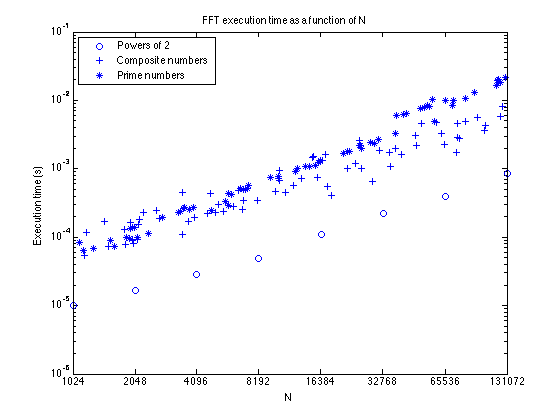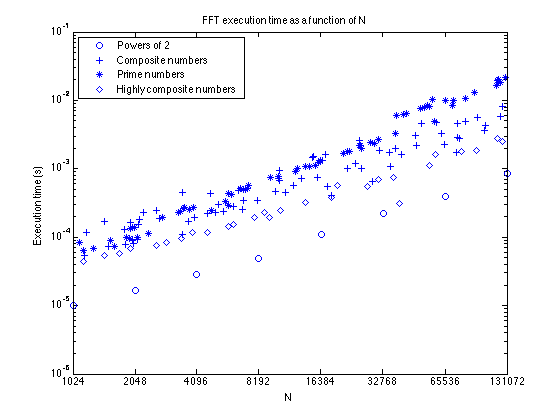# Timing the FFT

I've seen two questions recently about the speed of the fft function in MATLAB. First, a tech support question was forwarded to development. The user wanted to know how to predict the computation time for an FFT of a given length, N. This user was interested in values of N in the neighborhood of 4096 (2^12).

The second was a post in the MATLAB newsgroup comp.soft-sys.matlab. This user wondered why padding to the next power of two wasn't always the fastest way to compute the FFT.

Inspired by these questions, I want to show you today how to do some FFT benchmarking in MATLAB.

It turns out that, in general, the time required to execute an N-point FFT is proportional to N*log(N). For any particular value of N, though, the execution time can be hard to predict and depends on the number of prime factors of N (very roughly speaking). The variation in time between two close values of N can be as much as an order of magnitude.

Whenever I do FFT benchmarking, I find it very helpful to look at three sets of numbers:

• Powers of 2
• Composite numbers that are not powers of 2
• Prime numbers

Also, I have learned to look at plots that are log scale in N and that have roughly the same number of test values within each octave (or doubling) of N.

Constructing sets of N values along these lines takes a little thought. Here's some code.

First, how many powers of 2 do we want to examine? Based on the customer questions I saw, I want to examine the range from 1024 (2^10) to 131072 (2^17).

low2 = 10;
high2 = 17;
n2 = 2.^(low2:high2);


Next, I want to pick 10 composite numbers and 10 prime numbers in between successive powers of 2. I'd like to pick the numbers "randomly," but I also want my experiment to be repeatable. To satisfy these seemingly contradictory constraints, I'll reset the MATLAB random number generator before beginning.

rng('default');

% Initialize the vectors holding the prime N's and composite N's.
np = [];
nc = [];

for m = low2:high2
k = (2^m):(2^(m+1));
kp = k(2:end-1);
isp = isprime(kp);
primes = kp(isp);
composites = kp(~isp);

% Use randperm to pick out 10 values from the vector of primes and 10
% values from the vector of composites.
new_np = primes(randperm(length(primes),10));
new_nc = composites(randperm(length(composites),10));

np = [np new_np];
nc = [nc new_nc];
end


Now let's use the function timeit to measure the execution time required to compute FFTs for all these values of N. (If you don't have a recent version of MATLAB that has timeit, you can get a version of it from the File Exchange.)

t2 = zeros(size(n2));
for k = 1:length(n2)
x = rand(n2(k),1);
t2(k) = timeit(@() fft(x));
end

tp = zeros(size(np));
for k = 1:length(np)
x = rand(np(k),1);
tp(k) = timeit(@() fft(x));
end

tc = zeros(size(np));
for k = 1:length(nc)
x = rand(nc(k),1);
tc(k) = timeit(@() fft(x));
end


Now do a loglog plot of all these times.

loglog(n2,t2,'o')
set(gca,'xtick',2.^(10:17))
xlim([2^10 2^17])

hold on

loglog(nc,tc,'+')
loglog(np,tp,'*')

hold off

legend({'Powers of 2','Composite numbers','Prime numbers'}, ...
'Location','NorthWest')
xlabel('N')
ylabel('Execution time (s)')
title('FFT execution time as a function of N')You can see that there's a wide spread of execution times for the values of N that are not powers of 2.

One thing I'm not seeing is what the MATLAB Newsgroup poster reported. That is, I don't see a non-power-of-2 time that's faster than the next highest power of 2.

So let's look a little harder for composite numbers that are faster than what we've seen so far. Specifically, I'm going to look for values of N with prime factors no bigger than 3.

nn = [];
for m = low2:high2
k = (2^m):(2^(m+1));
kp = k(2:end-1);

kp = kp(randperm(length(kp)));
nn_m = [];
for q = 1:length(kp)
if max(factor(kp(q))) <= 3
nn_m = [nn_m kp(q)];

if length(nn_m) >= 4
% We've found enough in this part of the range.
break
end
end
end

nn = [nn nn_m];
end


Measure execution times for these "highly composite" numbers.

tn = zeros(length(nn),1);
for k = 1:length(nn)
x = rand(nn(k),1);
tn(k) = timeit(@() fft(x));
end


Add the times to the plot.

hold on
loglog(nn,tn,'d')
hold off

legend({'Powers of 2','Composite numbers','Prime numbers', ...
'Highly composite numbers'},'Location','NorthWest')You can see that sometimes a non-power-of-2 can be computed very fast, faster than the next higher power of 2. In this experiment we found one such value of N: 39366. This number has 10 prime factors:

factor(39366)

ans =

2     3     3     3     3     3     3     3     3     3



I hope you enjoyed these experiments with FFT benchmarking. I can tell you from personal experience that it can turn into almost a full-time hobby!

Published with MATLAB® R2014a

|